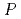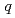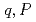An equation of power in subcritical quasi-steady state has been derived based on one-point kinetics equations for the purpose of utilizing it for the development of timely reactivity estimation from complicated time profile of neutron count rate. It linearly relates power,, to a new variable, which is a function of time differential of the power. It has been confirmed by using one-point kinetics code, AGNES, that the calculated points () are perfectly in a line described by the new equation and that points () calculated from transient subcritical experiments by using TRACY made a line with a slope indicated by the new equation.